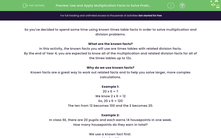# Use and Apply Multiplication Facts to Solve Problems

In this worksheet, students will be asked to use their times' table knowledge in order to solve other multiplication and division problems.This content is premium and exclusive to EdPlace subscribers.Key stage:  KS 2

Curriculum topic:   Exam-style Questions: SATs Maths

Curriculum subtopic:   Exam-Style Questions: Using Known Facts

Difficulty level:#### Worksheet Overview

So you've decided to spend some time using known times table facts in order to solve multiplication and division problems.

What are the known facts?

In this activity, the known facts you will use are times tables with related division facts.

By the end of Year 4, you are expected to know all of the multiplication and related division facts for all of the times tables up to 12.

Why do we use known facts?

Known facts are a great way to work out related facts and to help you solve larger, more complex calculations.

Example 1:

20 x 6 = ?

We know 2 x 6 = 12

So, 20 x 6 = 120

The ten from 12 becomes 100 and the 2 becomes 20.

Example 2:

In class 6E, there are 20 pupils and each earns 14 house points in one week.

How many house points do they earn in total?

We use a known fact first:

14 x 2 = 28

So, 14 x 20 = 280

The 20 from the 28 becomes 200 and the 8 becomes 80.

So, 14 x 20 = 280 house points.

Now it's over to you now to have a go at some practise questions.

Good luck!

### What is EdPlace?

We're your National Curriculum aligned online education content provider helping each child succeed in English, maths and science from year 1 to GCSE. With an EdPlace account you’ll be able to track and measure progress, helping each child achieve their best. We build confidence and attainment by personalising each child’s learning at a level that suits them.

Get started